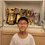# An extremely beautiful trigonometric pattern

There is a pattern which is quite common: $$\sin 0^\circ=\dfrac{\sqrt{0}}{2}, \sin 30^\circ=\dfrac{\sqrt{1}}{2}, \sin 45^\circ=\dfrac{\sqrt{2}}{2}, \sin 60^\circ=\dfrac{\sqrt{3}}{2}, \sin 90^\circ=\dfrac{\sqrt{4}}{2}$$

This pattern is useful because it helps us to memorize the trig functions of the main angles. However, when we start to look for the sine of some angles like $15^\circ$ or $67.5^\circ$, we may struggle for some time. However, there is a beautiful pattern: $\sin 0^{\circ} = \frac{1}{2}\sqrt{2-\sqrt{4}} \quad \sin 15^{\circ} = \frac{1}{2}\sqrt{2-\sqrt{3}} \quad \sin 22.5^{\circ} = \frac{1}{2}\sqrt{2-\sqrt{2}} \\ \sin 30^{\circ} = \frac{1}{2}\sqrt{2-\sqrt{1}} \quad \sin 45^{\circ} = \frac{1}{2}\sqrt{2\pm\sqrt{0}} \quad \sin 60^{\circ} = \frac{1}{2}\sqrt{2+\sqrt{1}} \\ \sin 67.5^{\circ} = \frac{1}{2}\sqrt{2+\sqrt{2}} \quad \sin 75^{\circ} = \frac{1}{2}\sqrt{2+\sqrt{3}} \quad \sin 90^{\circ} = \frac{1}{2}\sqrt{2+\sqrt{4}}$ That's amazing, isn't it? But I want to tell you, there's a reason behind. I'll show you that.

Firstly, from the first pattern, we know that: $\cos 0^\circ=\dfrac{\sqrt{4}}{2}\quad\cos 30^\circ=\dfrac{\sqrt{3}}{2}\quad\cos 45^\circ=\dfrac{\sqrt{2}}{2}\\\cos 60^\circ=\dfrac{\sqrt{1}}{2}\quad\cos 90^\circ=\dfrac{\sqrt{0}}{2}\quad\cos 120^\circ=-\dfrac{\sqrt{1}}{2}\\\cos 135^\circ=-\dfrac{\sqrt{2}}{2}\quad\cos 150^\circ=-\dfrac{\sqrt{3}}{2}\quad\cos 180^\circ=-\dfrac{\sqrt{4}}{2}$ Then, we'll use the half-angle formula $\sin^2 \dfrac{\theta}{2} = \dfrac{1-\cos\theta}{2}$

$\because 0^\circ \le\theta\le 180^\circ \rightarrow 0^\circ \le\dfrac{\theta}{2}\le 90^\circ \\ \therefore \sin \dfrac{\theta}{2} = \sqrt{\dfrac{1-\cos\theta}{2}}\ge 0 \\ \text{The }\cos\theta\text{ we want are all in the form }\pm\dfrac{\sqrt{n}}{2}\text{ where }n=0,1,2,3,4 \\ \quad\sin \dfrac{\theta}{2}=\sqrt{\dfrac{1\mp\frac{\sqrt{n}}{2}}{2}}=\sqrt{\dfrac{2\mp\sqrt{n}}{4}}=\dfrac{1}{2}\sqrt{2\mp\sqrt{n}}$

Therefore, we get the the result below: $\sin 0^\circ=\sin \dfrac{1}{2}\left(0^\circ\right)=\dfrac{1}{2}\sqrt{2-\sqrt{4}}\quad\sin 15^\circ=\sin \dfrac{1}{2}\left(30^\circ\right)=\dfrac{1}{2}\sqrt{2-\sqrt{3}}\quad\sin 22.5^\circ=\sin \dfrac{1}{2}\left(45^\circ\right)=\dfrac{1}{2}\sqrt{2-\sqrt{2}}\\\sin 30^\circ=\sin \dfrac{1}{2}\left(60^\circ\right)=\dfrac{1}{2}\sqrt{2-\sqrt{1}}\quad\sin 45^\circ=\sin \dfrac{1}{2}\left(90^\circ\right)=\dfrac{1}{2}\sqrt{2\mp\sqrt{0}}\quad\sin 60^\circ=\sin \dfrac{1}{2}\left(120^\circ\right)=\dfrac{1}{2}\sqrt{2+\sqrt{1}}\\\sin 67.5^\circ=\sin \dfrac{1}{2}\left(135^\circ\right)=\dfrac{1}{2}\sqrt{2+\sqrt{2}}\quad\sin 75^\circ=\sin \dfrac{1}{2}\left(150^\circ\right)=\dfrac{1}{2}\sqrt{2+\sqrt{3}}\quad\sin 90^\circ=\sin \dfrac{1}{2}\left(180^\circ\right)=\dfrac{1}{2}\sqrt{2+\sqrt{4}}$

I am so proud of myself that I can prove this beautiful pattern! (I first found this pattern when I see this) I hope it will help you guys when you are doing some work about trigonometry.Note by Isaac Yiu Math Studio
1 year, 9 months ago

This discussion board is a place to discuss our Daily Challenges and the math and science related to those challenges. Explanations are more than just a solution — they should explain the steps and thinking strategies that you used to obtain the solution. Comments should further the discussion of math and science.

When posting on Brilliant:

• Use the emojis to react to an explanation, whether you're congratulating a job well done , or just really confused .
• Ask specific questions about the challenge or the steps in somebody's explanation. Well-posed questions can add a lot to the discussion, but posting "I don't understand!" doesn't help anyone.
• Try to contribute something new to the discussion, whether it is an extension, generalization or other idea related to the challenge.
• Stay on topic — we're all here to learn more about math and science, not to hear about your favorite get-rich-quick scheme or current world events.

MarkdownAppears as
*italics* or _italics_ italics
**bold** or __bold__ bold
- bulleted- list
• bulleted
• list
1. numbered2. list
1. numbered
2. list
Note: you must add a full line of space before and after lists for them to show up correctly
paragraph 1paragraph 2

paragraph 1

paragraph 2

[example link](https://brilliant.org)example link
> This is a quote
This is a quote
    # I indented these lines
# 4 spaces, and now they show
# up as a code block.

print "hello world"
# I indented these lines
# 4 spaces, and now they show
# up as a code block.

print "hello world"
MathAppears as
Remember to wrap math in $$ ... $$ or $ ... $ to ensure proper formatting.
2 \times 3 $2 \times 3$
2^{34} $2^{34}$
a_{i-1} $a_{i-1}$
\frac{2}{3} $\frac{2}{3}$
\sqrt{2} $\sqrt{2}$
\sum_{i=1}^3 $\sum_{i=1}^3$
\sin \theta $\sin \theta$
\boxed{123} $\boxed{123}$

## Comments

Sort by:

Top Newest

But why the cos function follows that pattern?

- 1 year, 7 months ago

Log in to reply

I could only say, Mathematics is beautiful. It is just concidence

- 1 year, 7 months ago

Log in to reply

Really good and thanks for sharing this.

- 1 year, 7 months ago

Log in to reply

×

Problem Loading...

Note Loading...

Set Loading...